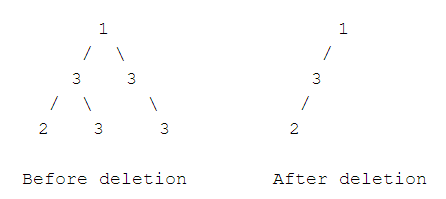New update is available. Click here to update.

# Delete Leaf Nodes With Value X

Last Updated: 26 Jul, 2020
Difficulty: Moderate

## PROBLEM STATEMENT

#### Your task is to delete all the leaf nodes with value X. In the process, if the newly formed leaves also have value X, you have to delete them too.

##### For example:

``````For the given binary tree, and X = 3:
``````##### Input Format:
``````The first line contains an integer T, the number of test cases. Then T cases follow.

For each test case, the first line contains input in the level order form. The input consists of values of nodes separated by a single space in a single line. In case a node is null, we take -1 in its place.

The second input line contains integer X, the value to be deleted.

For example, the input for the tree depicted in the below image would be :
````````````1
2 3
4 -1 5 6
-1 7 -1 -1 -1 -1
-1 -1
``````

#### Explanation :

``````Level 1 :
The root node of the tree is 1

Level 2 :
Left child of 1 = 2
Right child of 1 = 3

Level 3 :
Left child of 2 = 4
Right child of 2 = null (-1)
Left child of 3 = 5
Right child of 3 = 6

Level 4 :
Left child of 4 = null (-1)
Right child of 4 = 7
Left child of 5 = null (-1)
Right child of 5 = null (-1)
Left child of 6 = null (-1)
Right child of 6 = null (-1)

Level 5 :
Left child of 7 = null (-1)
Right child of 7 = null (-1)

The first not-null node (of the previous level) is treated as the parent of the first two nodes of the current level. The second not-null node (of the previous level) is treated as the parent node for the next two nodes of the current level and so on.

The input ends when all nodes at the last level are null (-1).
``````
##### Note :
``````The above format was just to provide clarity on how the input is formed for a given tree.

The sequence will be put together in a single line separated by a single space. Hence, for the above-depicted tree, the input will be given as:

1 2 3 4 -1 5 6 -1 7 -1 -1 -1 -1 -1 -1
``````
##### Output Format:
``````For each test case, in a new output line, print the inorder traversal of the tree.
``````
##### Note:
``````You don't need to print anything. Just make the changes in the given tree itself and return the root of the updated tree.
``````
##### Constraints:
``````1 <= T <= 100
0 <= N <= 3*10^3
1 <= A <= 10^5
1 <= X <= 10^5

Time limit: 1sec
``````## Approach 1

We do a depth-first traversal to travel the tree :

• Base case: If the root is NULL, we will return NULL
• If the root's left is not NULL, then we will update the root's left by using recursion and deleting all leaf nodes in the left subtree
• If the root's right is not NULL, then we will update the root's right by using recursion and deleting all leaf nodes in the right subtree
• Finally, we will check whether the root itself is a leaf. If yes, we will return NULL, otherwise, we will return the root itself